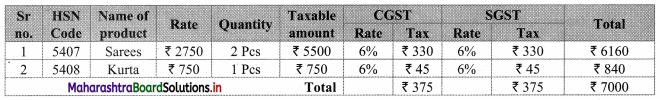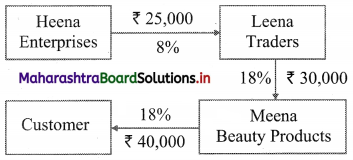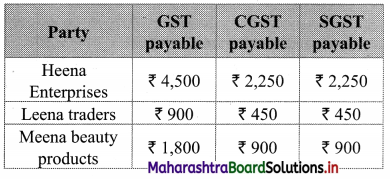# Maharashtra Board 11th Commerce Maths Solutions Chapter 9 Commercial Mathematics Ex 9.6

Balbharati Maharashtra State Board 11th Commerce Maths Solution Book Pdf Chapter 9 Commercial Mathematics Ex 9.6 Questions and Answers.

## Maharashtra State Board 11th Commerce Maths Solutions Chapter 9 Commercial Mathematics Ex 9.6

Question 1.
M/s Janaseva sweet mart sold sweets of ₹ 3,86,000. What CGST and SGST he will pay if the rate of GST is 5%?
Solution:
Given that M/s Janaseva sweet mart sold sweets of ₹ 3,86,000
∴ Bill amount = ₹ 3,86,000
GST payable at the rate 5%
∴ CGST and SGST applicable is 2.5% each
∴ CGST on the bill = $$\frac{2.5}{100}$$ × 3,86,000 = ₹ 9650
and SGST on the bill = $$\frac{2.5}{100}$$ × 3,86,000 = ₹ 9650

Question 2.
Janhavi Gas Agency purchased some gas cylinders for ₹ 5,00,000 and sold them to the customers for ₹ 5,90,000. Find the amount of GST payable and the amount of ITC. 5% GST is applicable.
Solution:
Given that, Janhavi Gas Agency purchased some gas cylinders for ₹ 5,00,000 and GST applicable is 5%.
∴ Input tax (ITC) = 5% of 5,00,000
= $$\frac{5}{100}$$ × 5,00,000
= ₹ 25,000
Janhavi Gas Agency sold the gas cylinders for ₹ 5,90,000
∴ Output tax for Janhavi Gas Agency = 5% of 5,90,000
= $$\frac{5}{100}$$ × 5,90,000
= ₹ 29,500
GST payable = Output tax – Input tax (ITC)
= 29,500 – 25,000
= ₹ 4500
∴ GST payable for Janhavi Gas Agency is ₹ 4,500 and ITC is ₹ 25,000.Question 3.
A company dealing in mobile phones purchased mobile phones worth ₹ 5,00,000 and sold the same to customers at ₹ 6,00,000. Find the amount of ITC and amount of GST if the rate of GST is 12%.
Solution:
Given that the rate of GST applicable is 12%.
The company purchased mobile phones worth ₹ 5,00,000.
∴ Input tax (ITC) = 12% of 5,00,000
= $$\frac{12}{100}$$ × 5,00,000
= ₹ 60,000
The company dealing in mobile phones sold the same to customers at ₹ 6,00,000.
∴ Output tax of the company = 12% of 6,00,000
= $$\frac{12}{100}$$ × 6,00,000
= ₹ 72,000
GST payable for the company = Output tax – Input tax (ITC)
= 72,000 – 60,000
= ₹ 12,000
∴ The ITC for the company is ₹ 60,000 and GST payable is ₹ 12,000.

Question 4.
Prepare business to customers (B2C) tax invoice using given information. Write the name of supplier, address, state, Date, Invoice Number, GSTIN etc. as per your choice
Supplier: ___________
State: ___________
Date: ___________
Invoice No: ___________
GSTIN: ___________
Particular: Rate of Sarees – ₹ 2750
Rate of GST 5% HSN 5407 – 2 pcs
Rate of Kurta – ₹ 750
Rate of GST 12% HSN 5408
Solution:
Supplier: M/s Swaglife Fashions
Address: 143, Shivaji Rasta, Mumbai 400001
Mobile No. 9263692111
Email: abc@gmail.com
State: Maharashtra
Date: 31/08/19
Invoice No: GST/110
GSTIN: 27ABCDE1234HIZS∴ Rate of 1 saree = ₹ 2750
∴ Rate of 2 sarees = 2 x 2750 = ₹ 5500
∴ GST on sarees = 12% of 5500
= $$\frac{12}{100}$$ × 5500
= ₹ 660
∴ CGST = SGST = ₹ 330
∴ Rate of 1 Kurta = ₹ 750
∴ GST on Kurta = 12% of 750
= $$\frac{12}{100}$$ × 750
= ₹ 90
∴ CGST = SGST = ₹ 45Question 5.
Heena Enterprise sold cosmetics worth ₹ 25,000 to Leena traders, a retailer. Leena Traders sold it further to Meena Beauty Products for ₹ 30,000. Meena Beauty Product sold it further to the customers for ₹ 40,000. The rate of GST is 18%. Find
(i) GST Payable by each party
(ii) CGST and SGST
Solution:∴ Output tax for Heena Enterprises = 18% of 25,000
= $$\frac{18}{100}$$ × 25,000
= ₹ 4,500
∴ GST payable by Heena Enterprises
Now output tax for Leena traders = 18% of 30,000
= $$\frac{18}{100}$$ × 30,000
= ₹ 5,400
∴ GST payable by Leena traders = Output tax – Input tax
= 5,400 – 4,500
= ₹ 900
∴ Output tax for Meena beauty products = 18% of 40,000
= $$\frac{18}{100}$$ × 40,000
= ₹ 7,200
∴ GST payable by Meena beauty products = Output tax – Input tax
= 7,200 – 5,400
= ₹ 1,800

(ii) Now, CGST = SGST = $$\frac{\text { GST }}{2}$$ = 9%
∴ Statement of GST payable at each stage can be tabulated as:Question 6.
‘Chitra furnishings’ purchased tapestry (curtain cloth) for ₹ 28,00,000 and sold for ₹ 44,80,000. Rate of GST is 5%. Find
(i) Input Tax
(ii) Output Tax
(iii) ITC
(iv) CGST and SGST
Solution:
Given, that ‘Chitra furnishings’ purchased tapestry (curtain cloth) for ₹ 28,00,000 and rate of GST is 5%
(i) Input tax = 5% of 28,00,000
= $$\frac{5}{100}$$ × 28,00,000
= ₹ 1,40,000
The tapestry was sold at ₹ 44,80,000

(ii) Output tax = 5% of 44,80,000
= $$\frac{5}{100}$$ × 44,80,000
= ₹ 2,24,000

(iii) Now ITC = Input tax = ₹ 1,40,000
GST payable = Output tax – ITC
= 2,24,000 – 1,40,000
= ₹ 84,000

(iv) CGST = SGST = $$\frac{\text { GST Payable }}{2}$$
= $$\frac{84,000}{2}$$
= ₹ 42,000
∴ CGST = SGST = ₹ 42,000Question 7.
Two friends ‘Aditi’ and ‘Vaishali’ went to a restaurant. They ordered 2 Masala Dosa costing ₹ 90 each 2 coffee costing ₹ 60 each and 1 sandwich costing ₹ 80. GST is charged at 5%. Find the Total amount of the bill including GST.
Solution:
Aditi and Vaishali ordered for 2 Masala Dosas, 2 Coffees and 1 Sandwich
∴ Total price of their order = 2 × 90 + 2 × 60 + 80 = ₹ 380
GST is charged at 5%
∴ GST on the total order = 5% × 380
= $$\frac{5}{100}$$ × 380
= ₹ 19
∴ Total bill amount including GST = 380 + 19 = ₹ 399